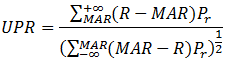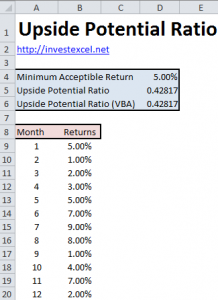Upside Potential Ratio – Spreadsheet and Guide

This article introduces the Upside Potential Ratio, a risk-adjusted investment performance benchmark.It also presents two methods of calculating this performance benchmark in Excel, one using a worksheet formula and another using VBA.

Several performance benchmarks use the standard deviation of the returns as a proxy for investment risk. The Sharpe Ratio, for example, is equal to the effective return divided by the standard deviation of the returns.

Standard deviation, however, penalizes both upside variation and downside variation equally. This does not match the risk preferences of most investors. Generally, investors want to encourage upside variation, but penalize downside variation.

The Sortino Ratio partially addresses this failing of the Sharpe Ratio. It is the effective return divided by the downside deviation. The Sortino Ratio favors investments with the highest return but the least downside volatility.

The Upside Potential Ratio is a further refinement that better addresses the risk preferences of investors. It is equal to the variation of the returns above a minimum acceptable return divided by the variation of the returns below a minimum acceptable returns. This favors investments with stable growth above a minimum acceptable return.

It is is defined by this equation.• MAR is the minimum acceptable return, and is chosen to match the investor’s goals
• R are the empirical investment returns
• Pr is the probability of making that return

The numerator is the first order higher partial moment. The denominator is the square root of the second order lower partial moment.Upside Potential Ratio in Excel

Assuming the empirical returns are in cells B8:B19 and the minimum acceptable return is in cell D4, the Upside Potential Ratio is given by this Excel matrix formula

=SUM(IF(B8:B19>D4,B8:B19-D4))*1/COUNT(B8:B19)/
SQRT(SUM(IF(B8:B19<D4,(B8:B19-D4)^2)*1/COUNT(B8:B19)))

This formula must be entered with CTRL+SHIFT+ENTER.

Upside Potential Ratio in VBA

Function UpsidePotentialRatio(returns As Range, MAR As Double) As Variant
Dim n As Integer
Dim i As Integer
Dim upside As Double
Dim downside As Double
n = returns.Rows.Count
upside = 0
downside = 0

For i = 1 To n
If returns(i) > MAR Then
upside = upside + (returns(i) - MAR) * (1 / n)
End If

If returns(i) < MAR Then
downside = downside + (returns(i) - MAR) ^ 2 * (1 / n)
End If
Next i
If downside > 0 Then
UpsidePotentialRatio = upside / Sqr(downside)
Else
UpsidePotentialRatio = "undefined"
End If
End Function3 thoughts on “Upside Potential Ratio – Spreadsheet and Guide”

1. I have a doubt. while the 12 data points are monthly numbers, and the MAR is usually a annualized number.

Is it the assumption of this example that MAR is 5% every month ??

Thanks

Vinod